Courses

# Quantitative Aptitude - Test 10

## 25 Questions MCQ Test SSC CGL Tier 1 Mock Test Series | Quantitative Aptitude - Test 10

Description
This mock test of Quantitative Aptitude - Test 10 for SSC helps you for every SSC entrance exam. This contains 25 Multiple Choice Questions for SSC Quantitative Aptitude - Test 10 (mcq) to study with solutions a complete question bank. The solved questions answers in this Quantitative Aptitude - Test 10 quiz give you a good mix of easy questions and tough questions. SSC students definitely take this Quantitative Aptitude - Test 10 exercise for a better result in the exam. You can find other Quantitative Aptitude - Test 10 extra questions, long questions & short questions for SSC on EduRev as well by searching above.
QUESTION: 1

### A cistern 5 m long and 8 m wide, contains water up to depth of 2 m 45 cm. Find the total area of wet surface.

Solution:

A cistern 5 m long and 8 m wide, contains water up to depth of 2 m 45 cm.
Here, cistern is in cuboid form
Length of cistern = 5 m
Breadth of cistern = 8 m
Height of cistern upto which its wet = 2 m 45 cm = 2.45 m
Total area of wet surface = {2(length + breadth) × height} + (length × breadth)
= {2(5 + 8) × 2.45} + (5 × 8)
= 63.7 + 40
=103.7 m2

QUESTION: 2

### In a triangle ABC, ∠B = 90°, D is a point on line AB such that it divides AB into two equal parts. AB is denoted by 2x and AC by y, then find the value of sin θ where ∠DCB = θ°.

Solution: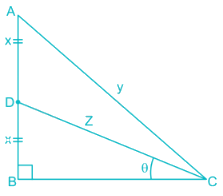Appling Pythagoras theorem on triangle ABC
AC2 = AB2 + BC2
⇒ y2 = (2x)2 + BC2
⇒ BC2 = y2 – 4x2
Now applying Pythagoras theorem on triangle DBC
DC2 = DB2 + BC2
⇒ z2 = x2 + BC2
⇒ BC2 = z2 – x2
Now, on comparing the value of BC2 we get
y2 – 4x2 = z2 – x2
∴ z2 = y2 – 3x2

∴ z = √(y2 – 3x2)
Now in Triangle DBC,
sin θ = DB/DC
∴ sin θ = x/z
∴ sin θ = x/√(y2 – 3x2)

QUESTION: 3

### The area of a circle is proportional to the square of its radius. A small circle of radius 3 cm is drawn within a larger circle of radius 5 cm. find the ratio of the area of the annular zone to the area of the larger circle. (Area of the annular zone is the difference between the area of the larger circle and that of the smaller circle).

Solution:

Area of a circle = π(radius)2
A small circle of radius 3 cm is drawn within a larger circle of radius 5 cm.
Area of large circle = π(5)2
= (22/7) × (5)2
= (22 × 5 ×5)/7
= 550/7
Area of small circle = π (3)2
= (22 × 3 × 3)/7
= 198/7
Area of annular zone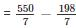= 352/7
The ratio of the area of the annular zone to the area of the larger circle = area of annular zone: area of large circle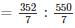= 16: 25

QUESTION: 4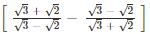simplifies to

Solution:

Given expression is,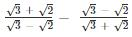Rationalising, we get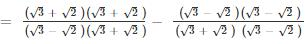Using identity: a2 – b2 = (a – b)(a + b) and a2 + b2 = a2 + 2ab + b2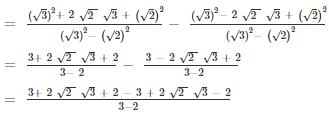= 4 × √2 × √3
= 4√6

QUESTION: 5

If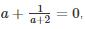then the value of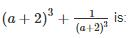Solution:

The given expression is: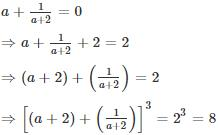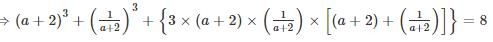[Using the formula (a + b)= a+ b+ 3ab(a + b)]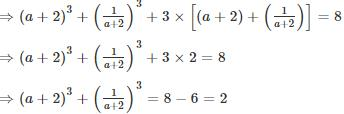Trick:
(a + 2) + 1/(a + 2) = 2
If x + 1/x = k then the value of x3 + 1/x3 will always be equal to (k3 – 3k)
Here k = 2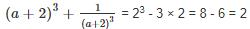QUESTION: 6

Directions: The production figure of a perfume manufactures are given in the form of percentage sub-divided bar diagram. Study the diagram and answer questions.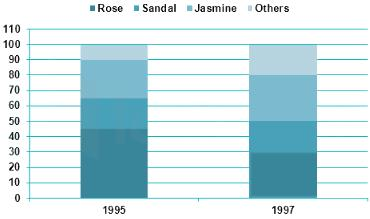Q. What is the production of jasmine perfume in the year 1997? Given that during the year 1997 total perfumed production was 5000 units.

Solution:

Given that during the year 1997 total perfumed production was 5000 units.
From the pie chart we can see, the percentage production of jasmine perfume in the year 1997 = (80 – 50) = 30% of total production.
∴ The production of jasmine perfume in the year 1997
= 30% of 5000
= 1500
∴ The production of jasmine perfume in the year 1997 = 1500

QUESTION: 7

Directions: The production figure of a perfume manufactures are given in the form of percentage sub-divided bar diagram. Study the diagram and answer questions.Q. What is the ratio of percentage production of rose perfume during 1995 to that during the year 1997?

Solution:

From the pie chart we can see, the percentage production of rose perfume in the year 1995 = (45 – 0) = 45% of total production.
Similarly, from the pie chart we can see, the percentage production of rose perfume in the year 1997 = (30 – 0) = 30% of total production.
The ratio of percentage production of rose perfume during 1995 to that during the year 1997 = 45: 30 = 3 : 2

QUESTION: 8

Directions: The production figure of a perfume manufactures are given in the form of percentage sub-divided bar diagram. Study the diagram and answer questions.Q. What is the percentage increase of % production of sandal perfume during the year 1995 over that during 1997?

Solution:

The percentage of production of sandal perfume during the year 1995 = (65 – 45) = 20%
The percentage of production of sandal perfume during the year 1997 = (50 – 30) = 20%
∴The percentage of production of sandal perfume during the year 1995 over that during 1997 = 0%

QUESTION: 9

From a cliff 120 m above the shore of a sea, the angle of depression of a ship is 60°. Find the distance from the ship to a point on the shore directly below the observer.

Solution: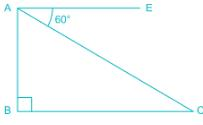From the diagram,
AB = height of the cliff = 120 m
Angle of depression = ∠ CAE = 60°
BC = Distance of the ship to the bottom of the cliff
∴ ∠ CAB = 90° - 60° = 30°
From ΔABC
tan ∠CAB = perpendicular/Base
⇒ tan 30° = BC/AB
⇒ 1/√3 = BC/120
⇒ BC = 120/√3 m
⇒ BC = 120√3/3 m = 40√3 m

QUESTION: 10

x = m sec α cos β, y = n sec α sin β , z = p tan α , then the value of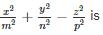Solution:

Given that,
x = m sec α cos β
Squaring both side we can write,
x2 = m2 sec2α × cos2 β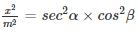Similarly we can write,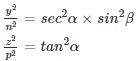Now we have to calculate the value of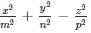Putting the values: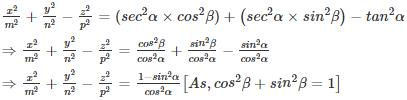QUESTION: 11

Sin232° + sin258° + cot258° is equal to

Solution:

Sinθ = cos(90° - θ) and sin2θ + cos2θ = 1 and Sec2θ = 1 + tan2θ
Sin232° + sin258° + cot258°
= sin232° + cos232° + cot258°
= 1 + cot258°
= cosec258°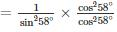= cot258°/cos258°
= cot258°/sin232°
= cosec232°cot258°

QUESTION: 12

If the arcs of same length in two circles subtend angles of 60° and 75° at their centres, the ratio of their radii is

Solution:

First circle subtend angle = 60°
2nd circle subtend angle = 75°
For the first circle:
⇒ angle1 = 60 = 60 × π/180 = π/3 radians
For the second circle:
⇒ angle2 = 75 = 75 × π/180 = 5 π/12 radians
We have the formula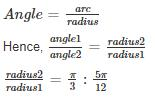QUESTION: 13

In Δ ABC, ∠A + ∠B = 65°, ∠B + ∠C = 140°, then find ∠B.

Solution:

We know that the sum of angles of a triangle is 180°.
∴ ∠A + ∠B + ∠C = 180°
Given: ∠A + ∠B = 65°
Replacing this value we get,
⇒ 65 + ∠C = 180
⇒∠C = 115°
Also, ∠B + ∠C = 140° (given)
⇒∠B + 115° = 140°
∴ ∠B = 25°

QUESTION: 14

If S is the circumcentre of ΔABC and ∠A = 50°, then the value of ∠BCS is

Solution:

Property of circumcentre
In any ΔXYZ, if O be circumcentre(where perpendicular bisectors of all three sides meet) of ΔXYZ.
Then, ∠XOZ = 2∠Y
∠ZOY = 2∠X
∠YOX = 2∠Z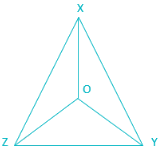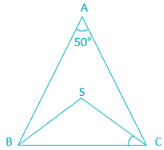Now, in ΔABC
S is the circumcentre of ΔABC and ∠A = 50°
∴ ∠BSC = 2∠A
= 2 × 50°
= 100°
Now in ΔBSC,
∠BCS = ∠CSB (as, BS and CS are radius of the circumcentre and angles opposite to the equal sides are equal)
∵ Sum of all angles of a triangle = 180°
∴ ∠BSC + ∠BCS + ∠CSB = 180°
⇒ 100° + 2∠BCS = 180°
⇒ 2∠BCS = 80°
⇒∠BCS = 40°

QUESTION: 15

A cistern is provided with two pipes A and B. A can fill it in 20 minutes and B can empty it in 30 minutes. If A and B be kept open alternately for one minute each, how soon will the cistern be filled?

Solution:

Given, A can fill it in 20 minutes and B can empty it in 30 minutes
Pipe A's work for one minute=1/20
Pipe B's work for one minute=1/30
∴ Total work done in two minute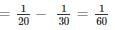In every 2 minute, 1/60th work is done, which means upto 114th minute, amount of work done will be = 57 × 1/60 = 57/60
Now the remaining work i.e. 3/60 (=1/20th) will be done by A in next 1 minute.
∴ Total time required = 114 + 1 = 115 minutes

QUESTION: 16

A cyclist completes a certain journey in 5 hours. He covers half the distance at 16 km./hr. and the second half of the distance at 24 km./hr. The distance (in km) covered by the cyclist is?

Solution:

Let,
Total distance covered = 2x km
We know that,
Time required = Distance covered/Speed
Given,
Half of the distance i.e. x km is covered at speed 16 km/hr
∴ Time required = x/16 hours
Next half of the distance i.e. x km is covered at speed 24 km/hr
∴ Time required = x/24 hours
Given,
Total time required = 5 hours
∴ x/16 + x/24 = 5
⇒ (3x + 2x)/48 = 5
⇒ 5x = 48 × 5
⇒ x = 48 km
∴ Total distance covered = 2 × 48 km = 96 km

QUESTION: 17

Ram bought 1600 eggs at the rate of Rs. 3.75 per dozen. He sold 900 of them at 2 eggs for Rs. 1 and the remaining at 5 eggs for Rs. 2. Find his loss or gain per cent.

Solution:

∵ Price of 12 eggs = Rs. 3.75
∵ Price of 1 egg = 3.75/12 = Rs.0.3125
∵ Price of 1600 eggs = Rs. (0.3125 × 1600) = Rs.500
∵ cost price, CP of eggs = Rs. 500
Selling price of 2 eggs = Rs. 1
∵ Selling price of 900 eggs =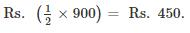Selling price of 5 eggs = Rs. 2
∴ Selling price of remaining (1600 - 900 = 700) eggs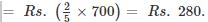∴ Total selling price, SP of eggs = Rs. 450 + 280 = Rs.730.
∵ SP > CP
∴ shopkeeper will have gain
Gain = SP – CP = Rs. 730 - 500 = Rs. 230.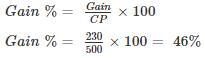Hence, Ram will have a gain percent of 46% by selling eggs.

QUESTION: 18

A 24 litres mixture contains milk and water in the ratio 5: 1. If 4 litres of water is mixed in the mixture, the percentage of milk in the new mixture is –

Solution:

Given,
Ratio of milk and water is 5: 1
Amount of mixture = 24 litres
∴ Amount of milk in the mixture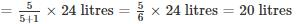∴ Amount of water in the mixture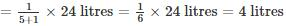If 4 litres of water is added
Amount of water in the new mixture = 4 + 4 = 8 litres
Total amount of mixture = 24 + 4 = 28 litres
And, Amount of milk in the new mixture = 20 litres
∴ Required percent
= (amount of milk/total amount in mixture) × 100%
= (20/28) × 100
= 500/7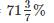QUESTION: 19

The average age of 11 players of a cricket team is increased by 2 months when two of them aged 18 years and 20 years are replaced by two new players. The average age of the new players is

Solution:

Formula:-
Sum of observations = average × number of observations
The average age of 11 players of a cricket team is increased by 2 months when two of them aged 18 years and 20 years are replaced by two new players
∴ Total age of players who are replaced= (18 +20) months
= 38 years
Age of 11 players of a cricket team is increased by (2 × 11= 22) months
Total Age of new players = 38years + 22 months
Average age of new players = (total age /no of players)
= (38years + 22 months)/2
= 19years 11 months

QUESTION: 20

The value of a machine depreciates every year at the rate of 10% on its value at the beginning of the year. If the current value of the machine is Rs.729, its worth 3 years ago was.

Solution:

Let, x be the value of the machine 3 years ago
Since, the value of machine depreciates at the rate 10% every year
∴ its value after 1 year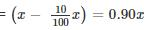Its value after 2 years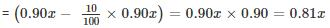Its value after 3 years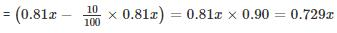According to the question,
0.729x = 729
x = 729/0.729 = 1000
Alternate:-
As we know that, if the value of machine depreciates by R% every year, then the value of machine after ‘t’ years will be:-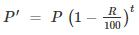Here, P’ = future value of machine
P = current value.
Here it is given that, current value is Rs. 729 and we will have to find out the value 3 years ago.
So, here P’ = 729 and P = ?, R = 10%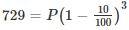⇒ P = 1000

QUESTION: 21

Simple interest on a certain sum is 16/25 of the sum. The rate per cent if the rate per cent and time (in years) are equal, is

Solution:

We know that, formula for simple interest: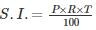Where,
S.I. = Simple Interest
P = principal
R = Rate of interest
T = Time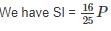Let R = T = k
Hence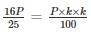1600 = 25 × k × k
64 = k2
k = +8 or k = -8
Since time period cannot be negative, hence, we have
k or rate of interest = 8 %

QUESTION: 22

The cost of a piece of diamond varies with the square of its weight. A diamond of Rs. 5,184 value is cut into 3 pieces whose weights are in the ratio 1 : 2 : 3. Find the loss involved in the cutting.

Solution:

Given, the cost of a piece of diamond varies with the square of its weight.
⇒ Price = k × weight2   (k is any constant)
Let the weight of a piece of diamond be ‘6x’.
Then, original price = k (6x)2 = 36kx2
⇒ 36kx2 = 5184      ……… eq(1)
The piece is cut into 3 pieces of weights ratio 1 : 2 : 3.
Now, the new price = k (x2 + (2x)2 + (3x)2) = 14kx2
From eq(1)
New price = (14 × 5184)/36 = 2016.
∴ The loss = 5184 – 2016 = Rs. 3168.

QUESTION: 23

Simplified value of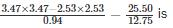Solution:

Follow BODMAS rule to solve this question, as per the order is given below,
Step - 1 - Parts of an equation enclosed in 'Brackets' must be solved first,
Step - 2 - Any mathematical 'Of' or 'Exponent' must be solved next,
Step - 3 - Next, the parts of the equation that contains 'Division' and 'Multiplication' are calculated,
Step - 4 - Last but not least, the parts of the equation that contains 'Addition' and 'Subtraction' should be calculated.
We know that,
(a2 – b2) = (a + b)(a – b)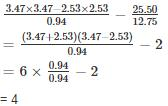QUESTION: 24

Convert 4.327327327.... into a rational expression.

Solution:

Let x = 4.327327327…. ………(1)
Multiply both sides by 1000
⇒ 1000x = 4327.327327… …….(2)
Subtract eq. (1) from eq. (2)
⇒ 999x = 4323
⇒ x = 4323/999

QUESTION: 25

461 + 462 + 463 + 464 is divisible by

Solution:

⇒ 461 + 462 + 463 + 464=461 (1 + 4 + 42 + 43)
⇒ 461 + 462 + 463 + 464=461 × 85
⇒ 461 + 462 + 463 + 464=461 × 5 × 17
So, the expression is divisible by 17